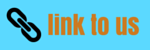# Motion

## 9. Uniform circular motion

• When an object moves in a circular path at a constant speed then motion of the object is called uniform circular motion.
• In our everyday life ,we came across many examples of circular motion for example cars going round the circular track and many more .Also earth and other planets revolve around the sun in a roughly circular orbits
• If the speed of motion is constant for a particle moving in a circular motion still the particles accelerates because of constantly changing direction of the velocity.
• If an object moves in a circular path with uniform speed, its motion is called uniform circular motion
• Here in circular motion, we use angular velocity in place of velocity we used while studying linear motion.
• Force which is needed to make body travel in a circular path is called centripetal force.
• We know that the circumference of a circle of radius $r$ is given by $2\pi r$. If the body takes $t$ seconds to go once around the circular path of radius $r$, the velocity $v$ is given by
$v = \frac{{2\pi r}}{t}$
• One thing we must keep in mind is that uniform linear motion is not accelerated but uniform circular motion is accelerated motion.

• Examples of uniform circular motion are
(a) Motion of artificial satellites around the earth
(b) Moon, the natural satellite of earth, moves in uniform circular motion round the earth.
(c) Cyclist moving on a circular track with a constant speed exhibits uniform circular motion.